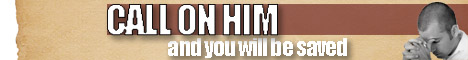Note:  Do not rely on this information. It is very old.

# Ellipsoid

Ellipsoid, one of the quadric surfaces, or surfaces such that any plane section gives a conic (q.v.). The true ellipsoid has three axes of symmetry at right angles to each other, each of different length. They meet at a point called the centre, which bisects them and all chords or diameters, as they are called, which are drawn through it and terminated by the surface. Any section of an ellipsoid is an ellipse, but there are two directions in which sets of circular sections may be obtained; the circle is regarded as a special case of ellipse. If a, b, and c are the semi-axes of an ellipsoid its volume will be -J it abc. If two of these are made equal a surface called a spheroid is obtained that may be generated by the rotation of an ellipse about either of its axes. If rotated about its major axis, a prolate spheroid is produced; if about its minor axis, an oblate spheroid. The shape of the earth is an instance of the latter. If all three axes be made equal the sphere is obtained, which must, therefore, be regarded as a special case of ellipsoid. All sections of a sphere are circular.